# Supplementary Documentation

Published February 27, 2019 by Scott Allen Burns; last updated Sept 30, 2020

Change Log
2/27/2019 Original publication.
8/1/2019 Added citation to published paper in Color Research & Application.
2/11/2020 Updated the symmetry section to expand on how it can be derived.
5/8/2020 Minor correction of location of update dates. Update of links to publications.
9/13/2020 Added section on Python implementation of the CAT and a link to that page.
9/30/2020 Modified source code presentation so that it can be copied and pasted.

### Introduction

This page contains supplementary documentation for the article “Chromatic Adaptation Transform by Spectral Reconstruction,” published in Color Research and Application in 2019 (Burns SA. Chromatic adaptation transform by spectral reconstruction. Color Res Appl. 2019;44(5):682-693). Any reference to “the Article” on this page refers to this document. The documentation that follows is organized according to the section numbers appearing in the Article. A preprint version of this paper is also available here.

Please note that the content of this page should not be considered peer reviewed by the journal, as it may be updated at any time. This page was last updated on the date shown above.

### 2. Examples of CATs Producing Negative Tristimulus Values

An instance of CAT02 producing a destination color with negative tristimulus values is presented here, specifically, for the case of Munsell 2.5G 4/8. The spectrophotometrically measured reflectance curve for this color is:

A purplish source illuminant is used, shown in the following table. Equal energy is used as the destination illuminant. Before applying CAT02, the source color tristimulus values must be obtained under the action of the illuminant. To do this, the 1931 CMFs are referenced to the source illuminant,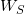:

Next, the source tristimulus values,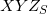, are computed from the reflectance curve for Munsell 2.5G 4/8,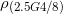, and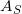:

(1)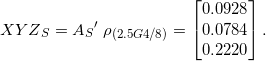Forming the CAT02 matrix starts with the transformation from tristimulus values to sharpened cone response space,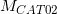:

(2)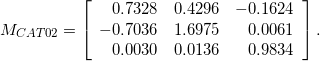The source and destination white points are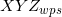= (2.145, 1, 4.729) and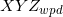= (1.000, 1.000, 1.000), which when pre-multiplied bygive white point cone responses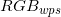= (1.2338, 0.2168, 4.6700) and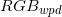= (1.000, 1.000, 1.000). The diagonal von Kries coefficient matrix (using full adaptation,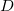=1) comprises ratios of destination to source white point cone responses:

(3)The final CAT02 matrix can now be computed as

(4)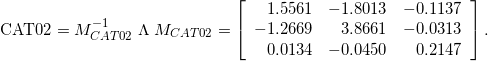The predicted destination color,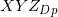, produced by CAT02 from the source tristimulus values,, ends up with negative tristimulus values:

(5)### 4. Chromatic Adaptation Transform by Spectral Reconstruction

Table 2 in the Article presents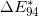color differences between the experimental corresponding-colors dataset destination colors,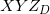, and the CAT-predicted destination colors,, for four methods: HPE, CAT02, CAT16, and the proposed CAT. In all cases, full adaptation (=1) is used.

To confirm the accuracy of these computations, they were compared to instances of the same computation by other authors in the literature, and were found to match exactly the published=1 values of: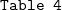of Reference: Susstrunk S, Holm J, Finlayson GD. Chromatic adaptation performance of different RGB sensors. Proc. SPIE 4300, Color Imaging: Device-Independent Color, Color Hardcopy, and Graphic Arts VI. Reiner Eschbach R, Marcu GG, Editors. 2001:172-183.of Reference: Bianco S, Schettini R. Two new von Kries based chromatic adaptation transforms found by numerical optimization. Color Res Appl. 2010;35(3):184-192.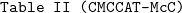of Reference: Hunt RWG, Li CJ, Juan LY, Luo MR. Further improvements to CIECAM97s. Color Res Appl. 2002;27(3):164-170.

Published=1 color difference values for CAT16 have not been found in the literature, for verification purposes.

### 5. Spectral Reconstruction Computation

Click here to open a text file containing a numerical example of the “spectral_recon” function shown in Section 5 of the Article, applied to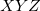= (0.7,0.6,0.5) using equal energy as the reference illuminant. It is best viewed by copying the text into an editor like Notepad and turning off word wrap. The values of each assignment statement on each line of the code are shown so it can be used to debug an implementation of the method. There may be small differences from one implementation to the next, mostly due to differences in floating point precision and different implementations of linear equation solving among the various utilities.

The author has also developed additional algorithms for reconstructing reflectance curves, including some that put an upper bound on the curve,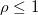. See this web page for more information on these additional methods and comparisons to natural paints and pigments, including Munsell color chips. An application of these spectral reconstruction methods for subtractive color mixture can be found here.

### 6. Implementing the CAT

Here are two MATLAB/Octave functions that implement the proposed CAT. Note that the matrix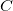used here (the finite-differencing constants array) is normally referred to asin other presentations concerning reflectance reconstruction. The switch toin the CAT presentation is due to the convention ofbeing used as the degree of adaptation.

The first function precomputes the necessary arrays for a given choice of source and destination illuminant white point tristimulus values (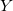should equal 1 in both).


% A is nx3 matrix of color matching functions (CMFs) by columns.
% XYZwps is 3x1 vector of source illuminant white point tristimulus values.
% XYZwpd is 3x1 vector of destination illuminant white point tristimulus values.
%        Note: XYZwps and XYZwpd should both have Y=1.
% C is nxn matrix of finite-differencing constants.
% As is nx3 matrix of Ws-referenced CMFs.
% Ad is nx3 matrix of Wd-referenced CMFs.
% Asd is nx3 matrix of Ws and Wd-referenced CMFs.
n=size(A,1);
C=full(gallery('tridiag',n,-2,4,-2));
C(1,1)=2;
C(n,n)=2;
Ws = spectral_recon(A,C,XYZwps); % See the Article for this function source code.
Wd = spectral_recon(A,C,XYZwpd); % See the Article for this function source code.
As=diag(Ws)*A;

 

The second function produces a predicted destination color, XYZdp, given a triplet of source tristimulus values, XYZs.


% Symmetric version of proposed CAT.
% C is nxn matrix of finite-differencing constants.
% As, Ad, and Asd are precomputed for a particular choice of
%     source and destination illuminants.
% As is nx3 Ws-referenced CMFs, As = diag(Ws)*A.
% Ad is nx3 Wd-referenced CMFs, As = diag(Wd)*A.
% Asd is nx3 Ws and Wd-referenced CMFs, Asd = diag(Ws)*Ad.
% XYZs is 3x1 vector of source tristimulus values.
% XYZdp is 3x1 vector of predicted destination tristimulus values.
rho=spectral_recon_sym(As,Asd,C,XYZs); % See the Article for this function source code.
XYZdp=XYZd*XYZs(2)/XYZd(2);

 

Here is an example:


>> % A is nx3 matrix of CMFs (1931 2 deg in this case).
>>
>> % Source illuminant A:
>> XYZwps = [1.0981; 1.0000; 0.3555];
>>
>> % Destination illuminant D65:
>> XYZwpd = [0.9501; 1.0000; 1.0882]
>>
>> % Precompute arrays for illum A and D65:
>>
>> % Three examples of CAT application:
XYZdp =
0.1699
0.3000
0.2415
XYZdp =
0.4393
0.5000
1.4401
XYZdp =
0.9501
1.0000
1.0882

 

The last example demonstrates that achromatic source colors map to achromatic colors with respect to the destination illuminant, as expected.

________________________________________

#### Python Implementation

All source code presented in the Article is written in the MATLAB/Octave language. Since publication of the Article, the CAT has been implemented in the Python programming language as well. This implementation is available here.

The Python implementation uses the SciPy package to implement the LAPACK routines for linear algebra. SciPy is in turn dependent on the NumPy package for numerical math. It is important to install the “Numpy+MKL” version of NumPy, which is linked to the Intel Math Kernel Library and includes required DLLs in the numpy.DLLs directory, and uses an optimized BLAS (Basic Linear Algebra Subprograms) library. The SciPy functions for linear algebra will solve MUCH faster this way.

### 7. Symmetry

To show how substituting some instances ofwith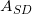makes the proposed CAT symmetric, we start with the simpler case of the non-log version of reflectance reconstruction, since it has a closed-form solution. The NLP being solved in this case is

(6)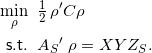See Section 3 of the Article for definitions of the symbols above. Again, note that the symbolis used here instead of the customaryfor the finite-differencing matrix because of the convention in CAT literature of usingto represent the degree of adaptation. The stationary conditions arising from the method of Lagrange multipliers are

(7)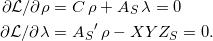Solving the first stationary condition for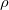gives

(8)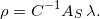Substituting this expression into the second stationary condition and solving for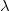yields

(9)Finally, substituting the expression forinto the previous equation gives us a closed-form solution for the spectral reconstruction:

(10)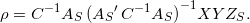To compute how this reconstructed reflectance would appear under a destination illuminant, we simply pre-multiplyby a destination-illuminant-referenced set of color matching functions,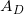:

(11)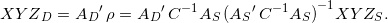This gives us a 3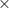3 CAT matrix of the form

(12)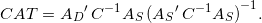Some comments are in order at this point.

1. Thematrix in the form as presented in the Article is singular, and so it is not suitable for this version of spectral reconstruction. However, a slight change to it, such as changing the first and last elements of the main diagonal to 4 instead of 2, makes the matrix invertible. The effect this change has on the reconstructed reflectance curve is to pull the ends of the curve to zero. This can be beneficial in some cases, as colored objects found in nature (e.g., common pigments; see Figure 8 in the Article) often have reflectances that drop to zero on the ultraviolet side, so the reconstruction is actually a better match on that end. The singularity ofwill not be an issue in the subsequent development, as it will not be inverted in the end result (Equations 8 and 9 in the Article).

2. The CAT derived above needs only to be computed once for a source/destination illuminant pair. Once this 33 CAT matrix is formed, each destination color computed from a source color requires only simple matrix multiplication, just as is the case with existing CATs. It will in fact perform very well when tested against the corresponding-color datasets. Unfortunately, it is capable of producing destination colors with negative tristimulus values, since the reconstructed reflectances can have negative values. The propensity for generating negative destination colors with this CAT is even more pronounced than it is with CAT02 and CAT16, and so it is not recommended for this reason. Nevertheless, it is a useful stepping stone in explaining the symmetric version of the method proposed in the Article.

Moving on to the issue of symmetry of the method, one way to express symmetry of the CAT is to describe mathematically the composite action of first transforming from source to destination illuminants, and then doing the reverse, transforming from destination to source illuminants. This composition should yield the identity matrix:

(13)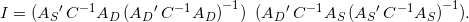By replacing two instances ofand two instances ofwith the dual-referencedwe get

(14)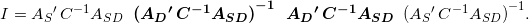The central pair of expressions (in bold) now cancel, and then the remaining two expressions also cancel, leaving the identity matrix, as desired. The author provides no other justification for the change toother than the observation that it simply works to make the CAT symmetric without any apparent negative side effects.

If we trace backwards to locate where the change tocould first have occurred, we find that if the stationary conditions are altered to be

(15)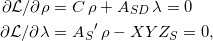then the symmetric form of the CAT is produced. This change only affects the Lagrange multiplier term in the first set of stationary conditions and does not alter the constraint expression,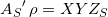, which is necessary to ensure that the reconstructed reflectance has the required-referenced tristimulus values.

Now turning to the log version of the spectral reconstruction used in the Article, an analogous treatment is applied to the first set of stationary conditions:

(16)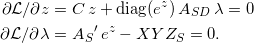This change will result in the symmetric version of the proposed method, as presented in Equations 12 and 13 of the Article.

UPDATE 2/11/2020
Back when the paper was published, I indicated that the earliest point at which the switch tocould occur was at the stationary conditions stage. I have since come to realize that it can be traced all the way back to a modified form of the original optimization statement. Considering the non-log version again, we replace

(17)with

(18)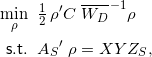The new term in the objective function is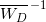, which is a diagonal matrix with the inverse of the destination illuminant on the main diagonal (and zeros elsewhere). If we construct the stationary conditions, we get

(19)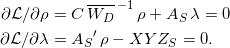Notice that if we multiply the first of these equations by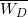, we have

(20)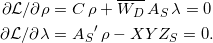The final step is to note that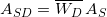, which transforms the equations above to the form shown in Equation 15. The remainder of the explanation of the symmetric CAT follows from Equation 15 above.

It is natural to ask how we should interpret the new objective function of Equation 18. One way to do this would be to absorb the illuminant term into theterm, yielding some sort of illuminant-weighted finite-differencing matrix. Perhaps a better way to express it would be to split the illuminant term and equally absorb it into the twoterms:

(21)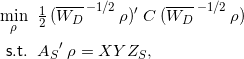The objective function can now be interpreted as a sum of squares of differences:

(22)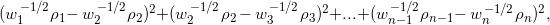whereis the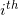term of illuminant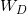. In the symmetric version of the CAT, we are seeking an illuminant-weighted smoothest (least slope squared) reflectance reconstruction to account for symmetry.

END OF UPDATE

Table 4 of the Article demonstrates the symmetric version in comparison to the original version: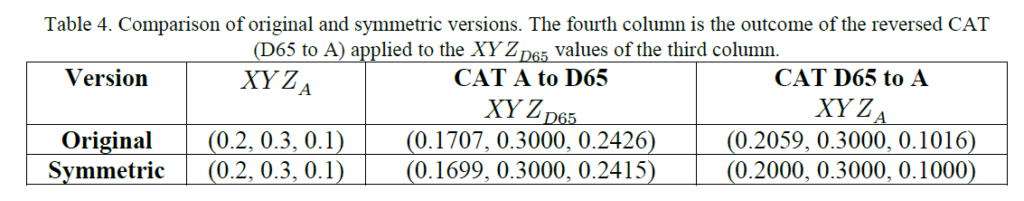See the Article for the meaning of the various columns. The entries in this table are produced by the following process.

First, the MATLAB/Octave function for reconstructing a reflectance curve for the original version is:


function rho=spectral_recon(As, C, XYZ)
% As is nx3 Ws-referenced CMFs, As = diag(Ws)*A
% C is nxn matrix of finite differencing constants (Eqn 5)
% XYZ is a 3x1 vector of target Ws-referenced tristimulus values
% rho is a nx1 vector of reflectance values (or zeros if failure)
n=size(As,1);
rho = zeros(n,1);
z=zeros(n,1);
lambda=zeros(3,1);
count=0;
maxit=20; % max number of iterations
ftol=1.0e-8; % solution tolerance
while count <= maxit
r=exp(z);
dr=diag(r);
v=dr*As*lambda;
F=[C*z+v; As'*r-XYZ];
J=[C+diag(v), dr*As; As'*dr, zeros(3)];
delta=J\(-F); % solve system of equations J*delta = -F
z=z+delta(1:n);
lambda=lambda+delta(n+1:n+3);
if all(abs(F) < ftol)
rho=exp(z);
return
end
count=count+1;
end

 

and the corresponding similar function for the symmetric case is:


function rho=spectral_recon_sym(As, Asd, C, XYZ)
% As is nx3 Ws-referenced CMFs, As = diag(Ws)*A
% Asd is nx3 Ws- and Wd-referenced CMFs, Asd = diag(Wd)*As
% C is nxn matrix of finite differencing constants
% XYZ is a 3x1 vector of target Ws-referenced tristimulus values
% rho is a nx1 vector of reflectance values (or zeros if failure)
n=size(As,1);
rho = zeros(n,1);
z=zeros(n,1);
lambda=zeros(3,1);
count=0;
maxit=20; % max number of iterations
ftol=1.0e-8; % solution tolerance
while count <= maxit
r=exp(z);
dr=diag(r);
v=dr*Asd*lambda;
F=[C*z+v; As'*r-XYZ];
J=[C+diag(v), dr*Asd; As'*dr, zeros(3)];
delta=J\(-F); % solve system of equations J*delta = -F
z=z+delta(1:n);
lambda=lambda+delta(n+1:n+3);
if all(abs(F) < ftol)
rho=exp(z);
return
end
count=count+1;
end

 

The main difference is the use of a dual-referenced set of CMFs in the symmetric version,. See the Article for definitions of all quantities. See the Article for MATLAB/Octave code to create matrix C.

The following MATLAB/Octave commands create the values in Table 4 for the non-symmetric case:


>> % Illuminant white points (A & D65)
>> XYZwps=[1.0981;1;0.3555]
XYZwps =
1.0981
1.0000
0.3555
>>
>> XYZwpd=[0.9501;1;1.0882]
XYZwpd =
0.9501
1.0000
1.0882
>>
>> % spectral reconstruction of illum
>> Ws=spectral_recon(A, C, XYZwps);
>> Wd=spectral_recon(A, C, XYZwpd);
>>
>> % source & dest illum-weighted CMFs
>> As=diag(Ws)*A;
>>
>> % source color
>> XYZs=[0.2;0.3;0.1]
XYZs =
0.2000
0.3000
0.1000
>>
>> % source spectral reconstruction
>> rho=spectral_recon(As, C, XYZs);
>>
XYZd =
0.2038
0.3581
0.2896
>>
>> % and output of proposed CAT
>> XYZdp=XYZd*(XYZs(2)/XYZd(2))
XYZdp =
0.1707
0.3000
0.2426
>>
>> % next apply CAT from dest->source
>> XYZs2=As'*rho2
XYZs2 =
0.1716
0.2500
0.0847
>>
>> XYZsp=XYZs2*(XYZdp(2)/XYZs2(2))
XYZsp =
0.2059
0.3000
0.1016
>>
>> % Note that XYZsp doesn't match XYZs

 

The symmetric case in the Table has values generated by the following MATLAB/Octave commands:


>> % Illuminant white points (A & D65)
>> XYZwps=[1.0981;1;0.3555]
XYZwps =
1.0981
1.0000
0.3555
>> XYZwpd=[0.9501;1;1.0882]
XYZwpd =
0.9501
1.0000
1.0882
>>
>> % spectral reconstruction of illuminant
>> Ws=spectral_recon(A, C, XYZwps);
>> Wd=spectral_recon(A, C, XYZwpd);
>>
>> % source & dest illum-weighted CMFs
>> As=diag(Ws)*A;
>>
>> % dual referenced CMFs
>> Asd=diag(Wd)*As;
>>
>> % source color
>> XYZs=[0.2;0.3;0.1]
XYZs =
0.2000
0.3000
0.1000
>>
>> % source spectral reconstruction
>> rho=spectral_recon_sym(As, Asd, C, XYZs);
>>
XYZd =
0.2033
0.3589
0.2890
>>
>> % and output of proposed symmetric CAT
>> XYZdp=XYZd*(XYZs(2)/XYZd(2))
XYZdp =
0.1699
0.3000
0.2416
>>
>> % next apply CAT from dest->source
>> XYZs2=As'*rho2
XYZs2 =
0.1672
0.2507
0.0836
>>